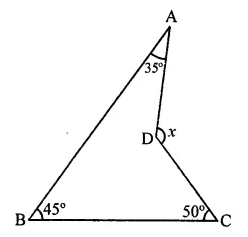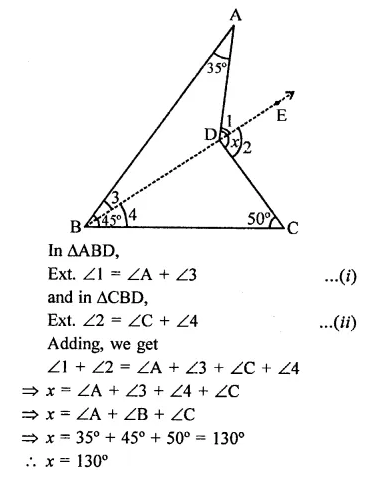• +91 9971497814
• info@interviewmaterial.com

# RD Chapter 11- Co-ordinate Geometry Ex-11.2 Interview Questions Answers

### Related Subjects

Question 1 : The exterior angles obtained on producing the base of a triangle both ways are 104° and 136°. Find all the angles of the triangle.

Answer 1 : In ∆ABC, base BC is produced both ways to D and E respectivley forming ∠ABE = 104° and ∠ACD = 136°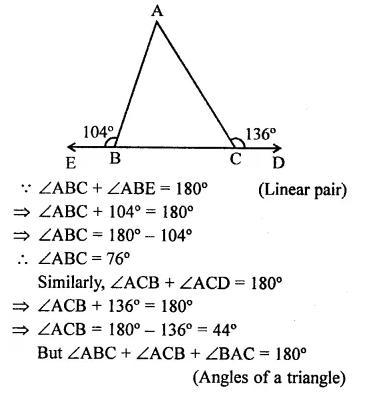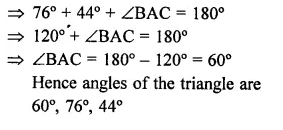Question 2 : In the figure, the sides BC, CA and AB of a ∆ABC have been produced to D, E and F respectively. If ∠ACD = 105° and ∠EAF = 45°, find all the angles of the ∆ABC.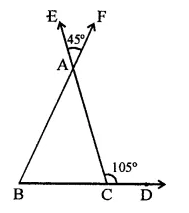In ∆ABC, sides BC, CA and BA are produced to D, E and F respectively.
∠ACD = 105° and ∠EAF = 45°
∠ACD + ∠ACB = 180° (Linear pair)
⇒ 105° + ∠ACB = 180°
⇒ ∠ACB = 180°- 105° = 75°
∠BAC = ∠EAF (Vertically opposite angles)
= 45°
But ∠BAC + ∠ABC + ∠ACB = 180°
⇒ 45° + ∠ABC + 75° = 180°
⇒ 120° +∠ABC = 180°
⇒ ∠ABC = 180°- 120°
∴ ∠ABC = 60°
Hence ∠ABC = 60°, ∠BCA = 75°
and ∠BAC = 45°

Question 3 : Compute the value of x in each of the following figures: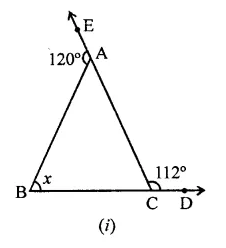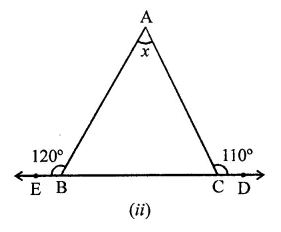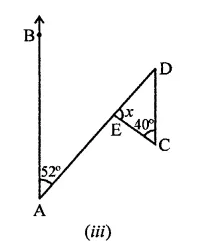Answer 3 : (i) In ∆ABC, sides BC and CA are produced to D and E respectively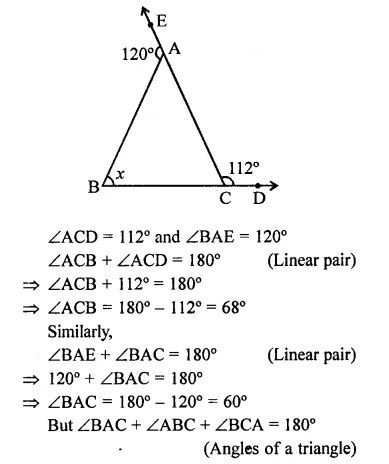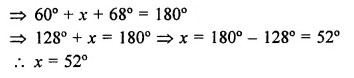(ii) In ∆ABC, side BC is produced to either side to D and E respectively
∠ABE = 120° and ∠ACD =110°
∵ ∠ABE + ∠ABC = 180° (Linear pair)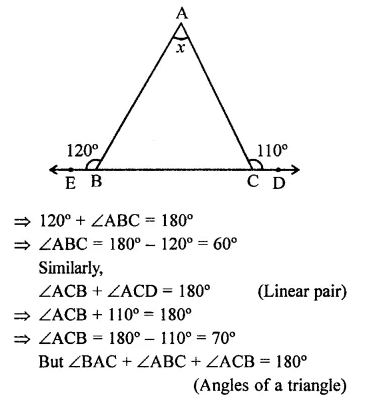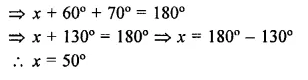(iii) In the figure, BA || DC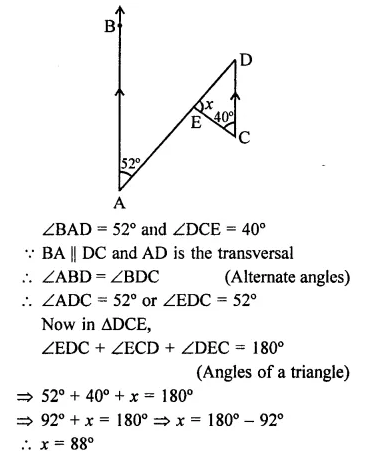Question 4 : In the figure, AC ⊥ CE and ∠A: ∠B : ∠C = 3:2:1, find the value of ∠ECD.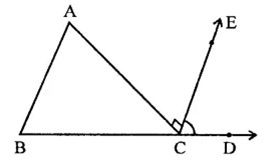In ∆ABC, ∠A : ∠B : ∠C = 3 : 2 : 1
BC is produced to D and CE ⊥ AC
∵ ∠A + ∠B + ∠C = 180° (Sum of angles of a triangles)
Let∠A = 3x, then ∠B = 2x and ∠C = x
∴ 3x + 2x + x = 180° ⇒ 6x = 180°
⇒ x =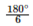= 30°
∴ ∠A = 3x = 3 x 30° = 90°
∠B = 2x = 2 x 30° = 60°
∠C = x = 30°
In ∆ABC,
Ext. ∠ACD = ∠A + ∠B
⇒ 90° + ∠ECD = 90° + 60° = 150°
∴ ∠ECD = 150°-90° = 60°

Question 5 : In the figure, AB || DE, find ∠ACD.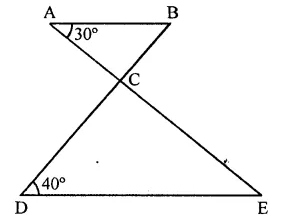In the figure, AB || DE
AE and BD intersect each other at C ∠BAC = 30° and ∠CDE = 40°
∵ AB || DE
∴ ∠ABC = ∠CDE (Alternate angles)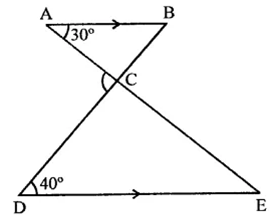⇒ ∠ABC = 40°
In ∆ABC, BC is produced
Ext. ∠ACD = Int. ∠A + ∠B
= 30° + 40° = 70°

Question 6 :
Which of the following statements are true (T) and which are false (F):
(i) Sum of the three angles of a triangle is 180°.
(ii) A triangle can have two right angles.
(iii) All the angles of a triangle can be less than 60°.
(iv) All the angles of a triangle can be greater than 60°.
(v) All the angles of a triangle can be equal to 60°.
(vi) A triangle can have two obtuse angles.
(vii) A triangle can have at most one obtuse angles.
(viii) If one angle of a triangle is obtuse, then it cannot be a right angled triangle.
(ix) An exterior angle of a triangle is less than either of its interior opposite angles.
(x) An exterior angle of a triangle is equal to the sum of the two interior opposite angles.
(xi) An exterior angle of a triangle is greater than the opposite interior angles.

(i) True.
(ii) False. A right triangle has only one right angle.
(iii) False. In this, the sum of three angles will be less than 180° which is not true.
(iv) False. In this, the sum of three angles will be more than 180° which is not true.
(v) True. As sum of three angles will be 180° which is true.
(vi) False. A triangle has only one obtuse angle.
(vii) True.
(viii)True.
(ix) False. Exterior angle of a triangle is always greater than its each interior opposite angles.
(x) True.
(xi) True.

Question 7 :
Fill in the blanks to make the following statements true:
(i) Sum of the angles of a triangle is ………
(ii) An exterior angle of a triangle is equal to the two …….. opposite angles.
(iii) An exterior angle of a triangle is always …….. than either of the interior opposite angles.
(iv) A triangle cannot have more than ………. right angles.
(v) A triangles cannot have more than ……… obtuse angles.

(i) Sum of the angles of a triangle is 180°.
(ii) An exterior angle of a triangle is equal to the two interior opposite angles.
(iii) An exterior angle of a triangle is always greater than either of the interior opposite angles.
(iv) A triangle cannot have more than one right angles.
(v) A triangles cannot have more than one obtuse angles.

Question 8 : In a ∆ABC, the internal bisectors of ∠B and ∠C meet at P and the external bisectors of ∠B and ∠C meet at Q. Prove that ∠BPC + ∠BQC = 180°.

Given : In ∆ABC, sides AB and AC are produced to D and E respectively. Bisectors of interior ∠B and ∠C meet at P and bisectors of exterior angles B and C meet at Q.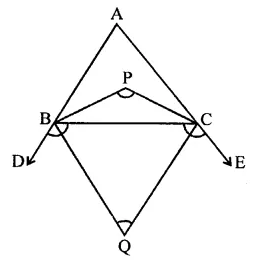To prove : ∠BPC + ∠BQC = 180°
Proof : ∵ PB and PC are the internal bisectors of ∠B and ∠C
∠BPC = 90°+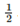∠A …(i)
Similarly, QB and QC are the bisectors of exterior angles B and C
∴ ∠BQC = 90° +∠A …(ii)
∠BPC + ∠BQC = 90° +∠A + 90° –∠A
= 90° + 90° = 180°
Hence ∠BPC + ∠BQC = 180°

Question 9 : In the figure, compute the value of x.